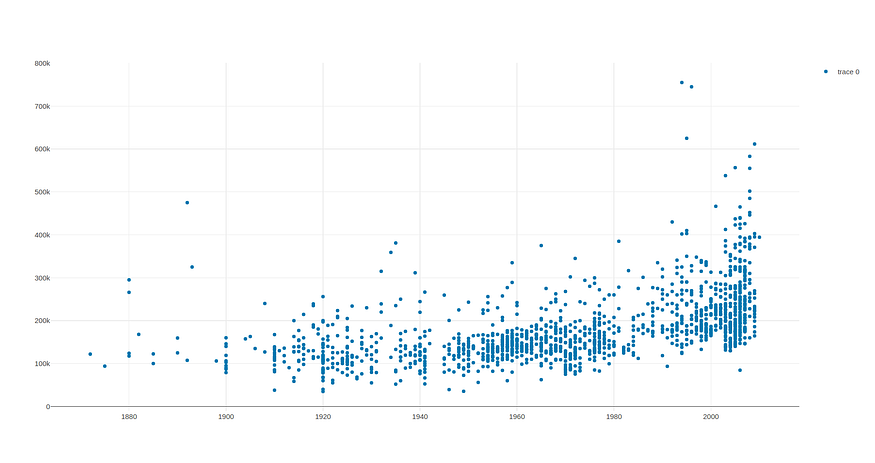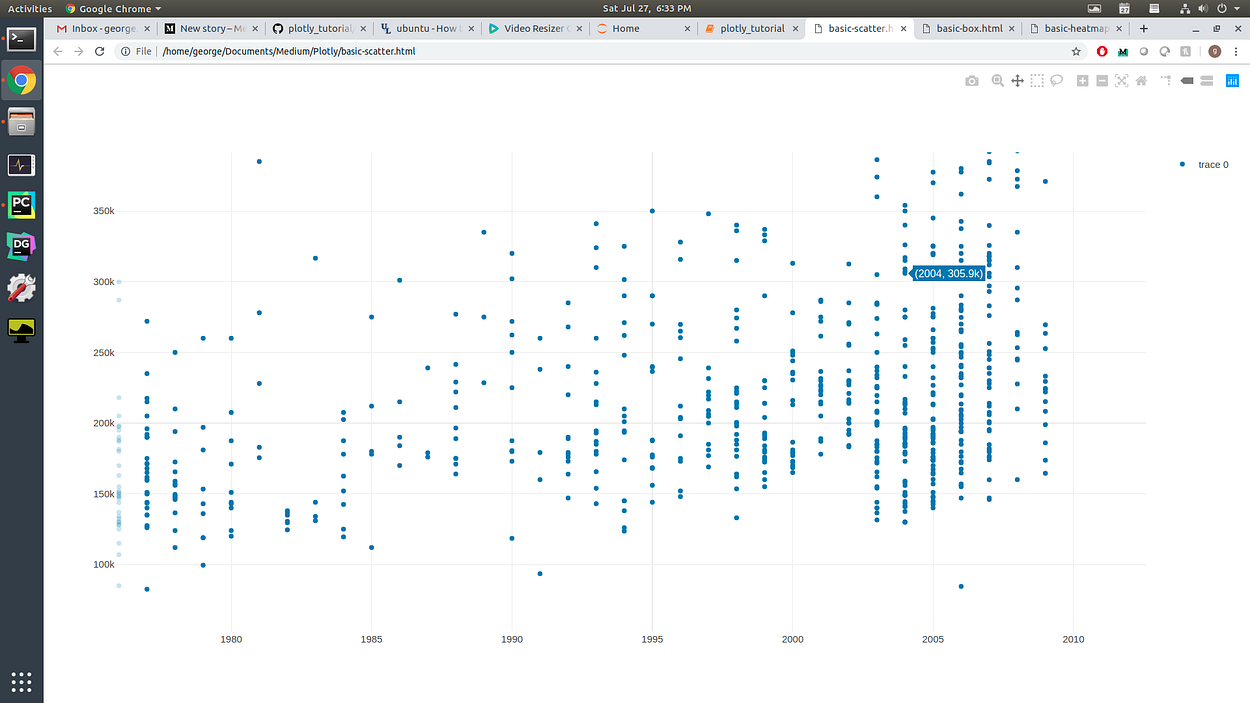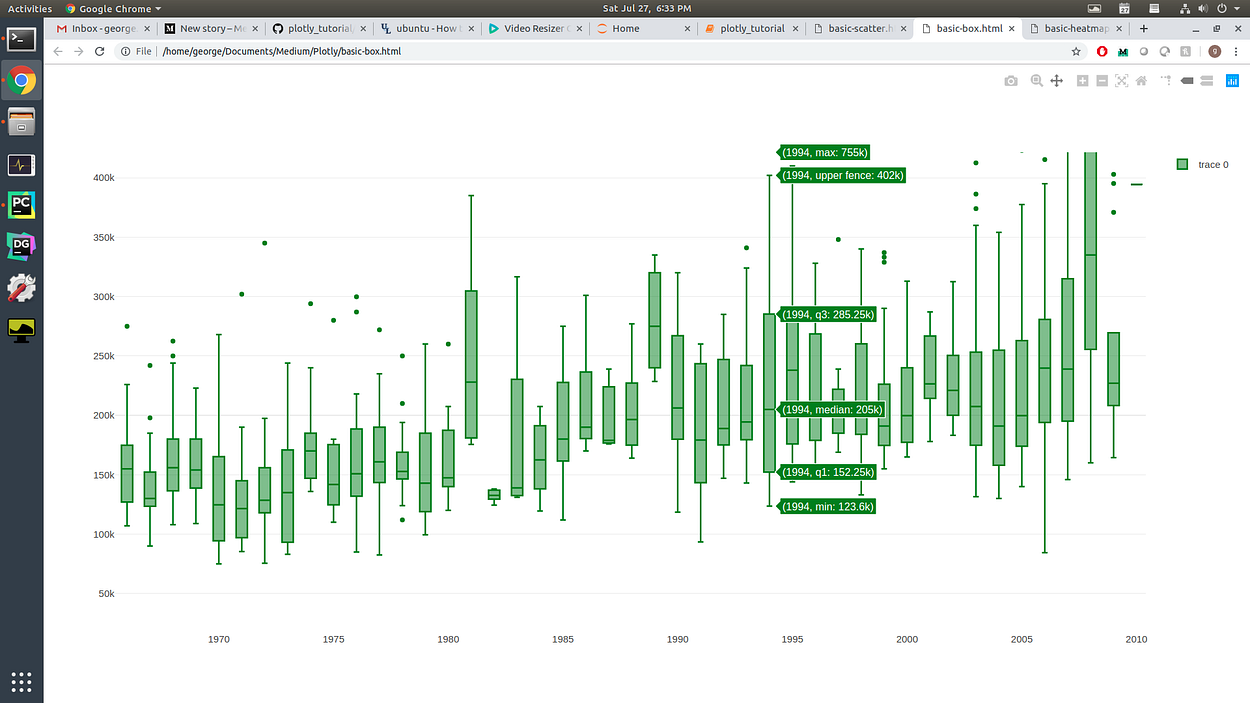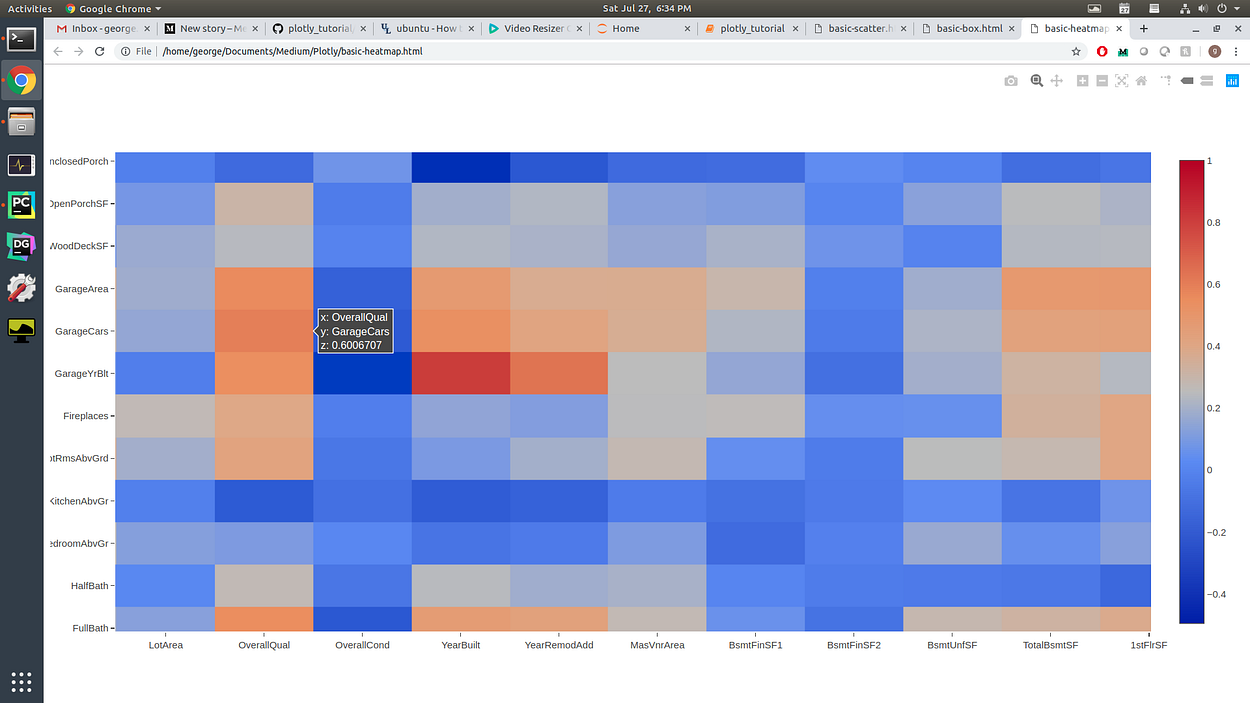#The Easy Way to Do Advanced Data Visualisation for Data Scientists

Creating effective data visualisations is a core skill for data scientists. This tutorial will guide you through how to easily develop interactive visualisations using the Python library plotly.commentsCreating effective data visualisations is one of the most valuable skills a Data Scientist can possess.

More than just making fancy charts, visualisation is a way of communicating a dataset’s information in a way that’s easy for people to understand. With a good visualisation, one can most clearly see the patterns and information that often lie hidden within the data.

In the early stages of a project, you’ll often be doing an Exploratory Data Analysis (EDA) to gain some insights into your data. Creating visualisations will help you along the way to speed up your analysis.

Towards the end of your project, it’s important to be able to present your final results in a clear, concise, and compelling manner that your audience, who are often non-technical stakeholders, can understand.

This article will be a tutorial on how to create fancy, advanced data visualisations — easily! We’ll be using plotly, a Python library for creating interactive visualisations!

### Introducing plotly

Plotly is an interactive, open-source, and browser-based graphing library for Python! It’s built on top of D3.js, making its visualisation capabilities much more far-ranging and flexible than the standard Matplotlib.

There are two main advantages to using Plotly over other Python libraries capable of plotting such as Matplotlib, Seaborn, and Pandas. These are:

(1) Ease of use — Creating interactive plots, 3D plots, and other complex graphics is just a few lines away. Doing the same thing in the other plotting libraries takes a lot more work.

(2) More capabilities — Since Plotly is built from D3.js, its plotting capabilities are much greater than other plotting libraries. Sun burst charts, candlestick charts, maps, and more are all possible with Plotly. See a full list here.

We can install Plotly with a single pip command:

```pip install plotly==4.0.0
```

For the rest of this tutorial, we’re going to be creating visualisations of the House Prices dataset which you can grab from Kaggle.

### Making Fancy Plots with Plotly

We can create some pretty fancy plots with Plotly!

To start, let’s import plotly and its internal graph objects component. We’ll also import pandas for loading up our dataset

```import plotly
import plotly.graph_objs as go

import pandas as pd
```

Reading in our dataset is of course just a one-liner in pandas:

```data = pd.read_csv('train.csv')
```

### Scatter Plots

For this exercise, we’re going to plot the SalesPrice vs. YearBuilt in a scatter plot. To do so, we’ll create a plotly Scatter graph object and put it into a trace.

```trace = go.Scatter(
x = data['YearBuilt'],
y = data['SalePrice'],
mode = 'markers',
showlegend = True
)
plot_data = [trace]
```

Then, plotting is just a single line away!

```plotly.offline.plot(plot_data, filename='basic-scatter')
```

The above command will open a new tab in your browser with the plot.Nothing fancy yet…. but just you wait!

Graph interactivity comes automatically built-in with Plotly. Check out the Jupyter Notebook to see what it can do! All of the commands are in the top-right of the browser:

• Hovering over each point shows the point’s x-y coordinates
• You can zoom in on a certain box of the data
• You can select and highlight certain points, either with a box or with Lasso
• You can pan around the entire plot to get a better look at things### Box Plots

This time we’ll do a box plot.

The process is about the same. We’ll create a graph object, put it into a trace, and then plot it in the browser:

```import plotly
import plotly.graph_objs as go
import pandas as pd
trace = go.Box(
x = data['YearBuilt'],
y = data['SalePrice'],
marker = {'color': 'green'},
showlegend = True,
)
plot_data = [trace]
plotly.offline.plot(plot_data, filename='basic-box')
```

Check out the Jupyter Notebook to see some of the fancy Plotly features with box plots!

By default, we get the same panning, zooming, and point selection. Since we now have a box plot, hovering over each box will show:

• Median
• 1st and 3rd quartiles
• Min and Max values of the data range
• The upper and / or lower fences if there are outliers### Heat Maps

Heat Maps are another powerful tool in any great Data Scientist’s toolbox. They’re especially effective for showing the relationships between multiple feature variables in one graph as well as the relative importance of each relationship.

To really show how your Heat Maps can be enhanced by using Plotly, we’re going to plot the correlation matrix of the House Prices dataset as a heat map. Plotting the correlation matrix of a dataset is a quick and easy way to get an overview of how your feature variables are influencing the target.

For plotly, we set the x and y to be the names of the columns, and the z to be the values in the matrix.

Plotting it all in our browser is, once again, is a walk in the park. You can try running it yourself using this Jupyter Notebook.

```import plotly
import plotly.graph_objs as go

import pandas as pd

corrmat = data.corr()

trace = go.Heatmap(z=corrmat, x=corrmat.columns.tolist(), y=corrmat.columns.tolist())

plot_data = [trace]

plotly.offline.plot(plot_data, filename='basic-heatmap')
```

Heatmaps in Matplotlib can be a bit tricky since you can’t see the exact value of each cell — you can only sort-of kind-of tell from the color. You could write code to make it interactive, but that’s quite the hassle in Matplotlib.

Plotly gives us interactivity out of the box, so when we plot a heat map we get a nice intuitive overview, but also have the option to check exact values if needed. The pan-and-zoom functionality of Plotly is also super clean, allowing for an easy way to do more detailed exploration, all from a visual point of view!Related: